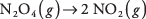## Gibbs Free Energy

The American physicist Josiah Gibbs introduced (ca. 1875) a thermo‐dynamic quantity combining enthalpy and entropy into a single value called free energy (or Gibbs free energy). In honor of its inventor, it is usually symbolized as G. The definition of free energy iswhere T is the temperature in Kelvin; entropies must be multiplied by temperature to get units of energy.

For studying chemical reactions, the relationship involves changes in the three thermodynamic quantities: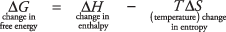A cardinal thermodynamic principle is that systems change toward minimum free energy. The sign of Δ G permits prediction of the behavior of a proposed chemical reaction with certainty.Although the free energy change of a reaction can be calculated from the preceding equation, if Δ H and Δ S for the reaction are not known, it is much more common to use this alternative equation:in which the values on the right are free energies of formation for each substance. For the standard state of 1 atm and 25°C, these values are called standard free energies of formation. As was the case with enthalpies of formation, the standard free energy of formation of an element is defined as zero. The value for each compound is the change in free energy associated with combining the elements to make one mole of the compound. In Table 1, the symbol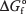has a superscript denoting standard and a subscript denoting formation.Given a listing of free energies of formation, the free energy change of a chemical reaction may be calculated in the same manner as you evaluated enthalpies of reaction and entropies of reaction. For the reaction that was discussed earlier,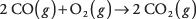the free energy of reaction is calculatedSince the change in free energy is negative, the forward reaction occurs spontaneously. Remember, systems change spontaneously to minimize free energy. Because the forward reaction loses free energy as it proceeds, that is the direction it will move spontaneously.

As another example of this important technique, consider the reaction involving oxides of both carbon and sulfur: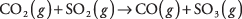From the free energies of formation given in Table 1, you can calculate the free energy of the reaction: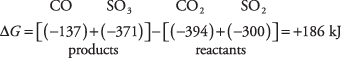This positive value for the free energy of reaction means that the reaction will not proceed to the right. Instead, the reverse reaction is favored, with CO and SO 3 reacting to yield CO 2 and SO 2. Moving to the left minimizes the free energy. Of course, this reaction can take place only if both carbon monoxide and sulfur trioxide are present initially. If you start with only carbon dioxide and sulfur dioxide, no reaction can occur.

The free energy calculations for both reactionsandare valid only at 25°C and 1 atm because standard free energies of formation are utilized to calculate the free energy changes of the two reactions.

However, if you know both the standard enthalpies of formation and standard entropies for every substance in a reaction, you can estimate the free energy of reaction at other temperatures by using the equation

Δ G = Δ HTΔ S

which, you may recall, is Gibbs's definition of the free energy change.

Use the preceding equation to estimate the free energy change at 400°C for the reactionYou need tabulated values of standard enthalpies of formation and standard entropies for the three gases, as shown in Table 2.Using the values in Table 2, you should be able to calculate the changes for the reaction at 25°C and obtain

Δ H = –566 kJ

Δ S = –173 J/deg

Perform both calculations now to make sure that you understand how they were done. Notice that the energy units for the values differ by a factor of 1,000; in the calculations that follow, Δ S is divided by 1,000 to make the terms comparable, converting calories to kilocalories.

Use these values now to calculate the free energy of reaction from the equation:

Δ G = Δ HTΔ S

At 25°C, which equals 298 K,

Δ G = (–566) – (298)(–0.173) = 514

At 400°C, which equals 673 K,

Δ G = (–566) – (673)(–0.173) = 450 kJ

The two results for the free energy of reaction differ because of the change in temperature. The first free energy change (at 25°C) is accurate because the tabulated values of enthalpies of formation and entropies are valid at that standard temperature. The second free energy change (at 400°C) is only a convenient estimate, based on the assumption that Δ H and Δ S do not change significantly with temperature. Actually, both do change slightly with temperature.

Table 3 provides information you should know about enthalpy, entropy, and free energy.Don't forget that both the calorie and the joule are units of energy in published charts, so you will often have to do a conversion to obtain the unit that you want.

• Use the chart of standard free energies of formation (Table 1) to determine the free energy change in the following reaction and report whether the reaction will proceed.• Use the standard values in Table 4 to compare the free energy change of the reaction at both 25°C and 100°C.